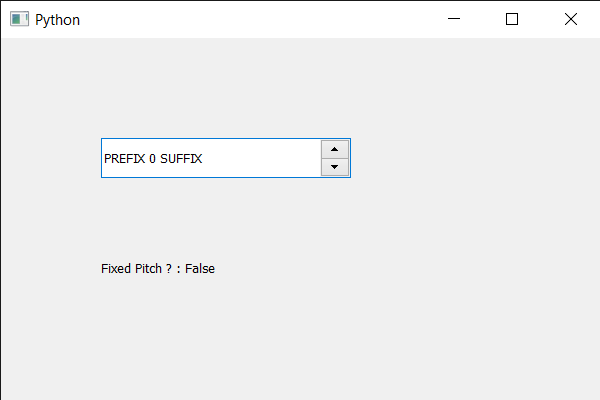# PyQt5 QSpinBox – Setting Hinting Preference

• Last Updated : 22 May, 2020

In this article we will see how we can check if the text of the spin box is fixed pitch or not, a mono-spaced font, also called a fixed-pitch, fixed-width, or non-proportional font, is a font whose letters and characters each occupy the same amount of horizontal space. This contrasts with variable-width fonts, where the letters and spacings have different widths.

In order to get the information about the font we have to get the QFontInfo object of spin box which can be done using `fontInfo` method.

In order to do this we fixedPitch method with the QFontInfo object of the spin box

Syntax : font_info.fixedPitch()

Argument : It takes no argument

Return : It returns bool

Below is the implementation

 `# importing libraries``from` `PyQt5.QtWidgets ``import` `*` `from` `PyQt5 ``import` `QtCore, QtGui``from` `PyQt5.QtGui ``import` `*` `from` `PyQt5.QtCore ``import` `*` `import` `sys`` ` ` ` `class` `Window(QMainWindow):`` ` `    ``def` `__init__(``self``):``        ``super``().__init__()`` ` `        ``# setting title``        ``self``.setWindowTitle(``"Python "``)`` ` `        ``# setting geometry``        ``self``.setGeometry(``100``, ``100``, ``600``, ``400``)`` ` `        ``# calling method``        ``self``.UiComponents()`` ` `        ``# showing all the widgets``        ``self``.show()`` ` `        ``# method for widgets``    ``def` `UiComponents(``self``):``        ``# creating spin box``        ``self``.spin ``=` `QSpinBox(``self``)`` ` `        ``# setting geometry to spin box``        ``self``.spin.setGeometry(``100``, ``100``, ``250``, ``40``)`` ` `        ``# setting range to the spin box``        ``self``.spin.setRange(``0``, ``999999``)`` ` `        ``# setting prefix to spin``        ``self``.spin.setPrefix(``"PREFIX "``)`` ` `        ``# setting suffix to spin``        ``self``.spin.setSuffix(``" SUFFIX"``)`` ` `        ` `        ``# creating a label``        ``label ``=` `QLabel(``self``)`` ` `        ``# setting geometry to the label``        ``label.setGeometry(``100``, ``200``, ``300``, ``60``)`` ` `        ``# getting the font info``        ``font_info ``=` `self``.spin.fontInfo()`` ` `        ``# getting fixed pitch status of text``        ``check ``=` `font_info.fixedPitch()`` ` `        ``# setting text to the label``        ``label.setText(``"Fixed Pitch ? : "` `+` `str``(check))`` ` ` ` `# create pyqt5 app``App ``=` `QApplication(sys.argv)`` ` `# create the instance of our Window``window ``=` `Window()`` ` `# start the app``sys.exit(App.``exec``())`

Output :My Personal Notes arrow_drop_up